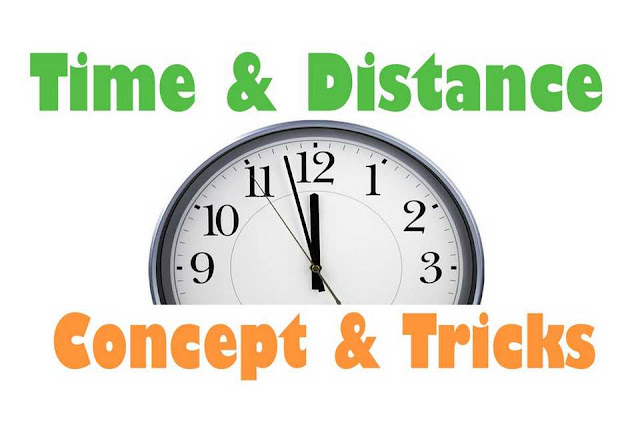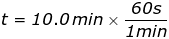## Time and Distance Formula

Aspirants who are going to appear in competitive exams should know the Time and Distance Formula. In quantitative aptitude section, candidates spend more time as they don’t know the formulas, shortcuts and short tricks to solve problems. So they find it very difficult but the fact is quantitative aptitude section is very easy. If you know the shortcuts, methods and short tricks then you can solve any problem in very less time. Competitive exams need accuracy and speed; if you have both of them then you can crack any tough exam.

Formula of distance, speed and time is correlated with each other, so don’t get confused among them. Aspirants can prepare from the sample papers and previous year question papers for the upcoming exams, you can buy it from the nearest books shop or download it from their official website. We the team of www.privatejobshub.in are providing some time and distance formulas and some useful short ticks below for your convenience. Take a look and read them carefully.

Methods & Short Tricks to Solve Problems

Generally students don’t know the basic tricks and shortcuts to solve the questions and that’s why they were unable to score good marks. Time and Distance formula and some shortcuts are available below so you can attempt all the questions and score better marks.

Formulas and how to convert them
Basics:Convert kilometers per hour (km/hr) to meters per second (m/s):

x km/hr = x X 5/18 m/s

Convert meters per second (m/s) to kilometers per hour (km/hr):

x m/s= x X 185 km/hr
Speed and relation between speed, distance and time

Speed is a measure of how quickly an object moves from one place to another. It is equal to the distance traveled divided by the time. Types of speed are mentioned below:

Average Speed:

If an object covers a certain distance at x kmph and an equal distance at y kmph, the average speed of the whole journey

=2xy/x+y kmph

Relation between Distance, Speed and Time:

Speed and time are inversely proportional (when distance is constant)

speed1/time (when distance is constant)

If the ratio of the speeds of A and B is a:b , a:b, then, the ratio of the time taken by them to cover the same distance is

1/a:1/b=b:a

Assume two objects A and B start at the same time in opposite directions from P and Q respectively. After passing each other, A reaches Q in a seconds and B reaches P in b seconds. Then,

Speed of A : Speed of B =√b:√a

An object covered a certain distance at a speed of v kmph. If it had moved v1 kmph faster, it would have taken t1 hours less. If it had moved v2 kmph slower, it would have taken t2 hours more. Then,Check This Also: How To Improve your IQ

Relative Speed:

If two objects are moving in the same direction at v1 m/s and v2 m/s respectively

where v1>v2, then their relative speed = (v1−v2) m/s

Consider two objects A and B separated by a distance of d meter. Suppose A and B start moving in the same direction at the same time such that A moves towards B at a speed of a meter/second and B moves away from A at a speed of b meter/second where a>b. Then,

relative speed =(a−b) meter/second

time needed for A to meet B =d/a−b seconds

If two objects are moving in opposite directions at v1 m/s and v2 m/s respectively, then their relative speed = (v1+v2) m/s

Consider two objects A and B separated by a distance of d meter. Suppose A and B start moving towards each other at the same time at a meter/second and b meter/second respectively. Then,

relative speed =(a+b) meter/second time needed for A and B to meet each other =d/a+b seconds

Want help in Preparation? Get Tips: Practice Which Helps In Preparation

Illustrations

1) A dog runs from one side of a park to the other. The park is 80.0 meters across. The dog takes 16.0 seconds to cross the park. What is the speed of the dog?

Answer: The distance the dog travels and the time it takes are given. The dog’s speed can be found with the formula:s = 5.0 m/s

The speed of the dog is 5.0 meters per second.

2) A golf cart is driven at its top speed of 27.0 km/h for 10.0 minutes. In meters, how far did the golf cart travel?

Answer: The first step to solve this problem is to change the units of the speed and time so that the answer found will be in meters, since this is what the question asks for. The speed is:

s = 27.0 km/hs = 7.50 m/s

Converting the units, the speed is 7.50 m/s. The time the cart traveled for was:

t = 10.0 mint = 600s

The speed of the cart and the time of travel are given, so the distance traveled can be found using the formula:

d = st
d = (7.50 m/s) (600 s)
d = 4500 m

The golf cart traveled 4500 m, which is equal to 4.50 km.

 Take a Test  Now

3) A person goes from A to B at the speed of 40 kmph and comes back at the speed of 60 kmph. What is his average speed for the whole journey?

Answer: Since the distance travelled on both sides is the same, we can use the formula of harmonic mean of speeds

Average Speed: 2xy/(x+y) where, x is the speed while going from A to B and y is the speed while coming back.

So using this formula, we get the answer as 48 kmph.

4) Moving at 50 kmph, a person reaches his office 10 min late. Next day, he increases his speed and moves at 60 kmph and reaches his office 5 min early. What is the distance from his home to his office?

Answer: We can observe that difference in timings on both days is 15 min (and not 5 min, as one day he is late and on the other day he is early)

Let the required distance = D km. As time taken at the speed of 50 kmph is more than time taken at 60 kmph, so equation can be formed as D/50-D/60=15/60 .

Solving this equation, we get the answer as 75 km.

Check Related Page Also:
Final words

Dear students, read all the time and distance formula, remember the shortcuts and methods at the time of examination. We hope that the above information that we provided it will be helpful for you. At last we wish to say all the best to the aspirants who are going to attend the exams.